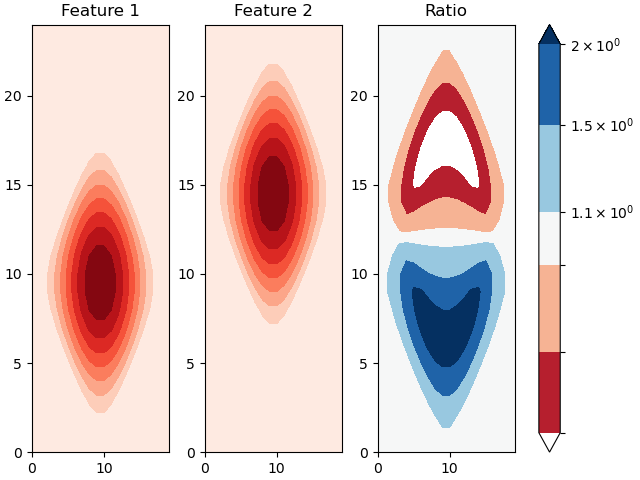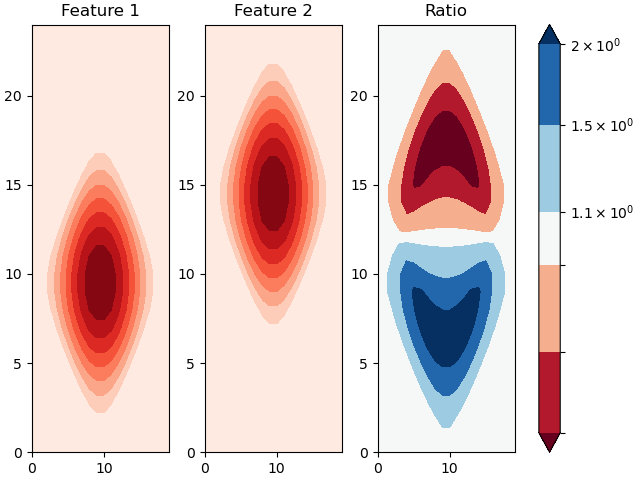# Contourf log-normed colours below lowest contour

I want to plot a contour of the ratio of two arrays, to show how a feature has moved. It seemed intuitive to set `norm="log"` to get sensible colours, and the plot almost looks right except that values below my lowest contour are white. The “under” value of the colormap is the expected dark red, so I guess these values are being marked as invalid. How can I make my lowest values dark red? Or is there a better way to do this?

I’m also curious about why the <1 values are not labelled on the colour bar (though this is not an issue in my real use-case).

``````import numpy as np
import matplotlib.pyplot as plt

y = np.linspace(-10, 10, 20)
x_2d, y_2d = np.meshgrid(y, y)

data = np.cos(x_2d * np.pi/10.) + np.cos(y_2d * np.pi/10.) + 1
np.place(data, data < 1., 1.)

data1 = np.ones((25, 20))
data2 = np.ones((25, 20))
data1[:20] = data
data2[-20:] = data
ratio = data1 / data2

fig, axs = plt.subplots(1, 3, layout="constrained")

axs.contourf(data1, cmap="Reds")
axs.set_title("Feature 1")

axs.contourf(data2, cmap="Reds")
axs.set_title("Feature 2")

ratio_contour = axs.contourf(ratio, cmap="RdBu", extend="both", norm="log",
levels=[1/2, 1/1.5, 1/1.1, 1.1, 1.5, 2])
plt.colorbar(ratio_contour, ax=axs)
axs.set_title("Ratio")

plt.savefig("test.png")

print(f"{ratio_contour.cmap.get_under()=}")
``````
``````ratio_contour.cmap.get_under()=array([0.40392157, 0.        , 0.12156863, 1.        ])
``````Never mind. I found it myself…

``````norm=mcolors.LogNorm(vmin=1/2, vmax=2, clip=True)
``````1 Like